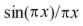### Possible connection between g(x) and Fibonacci numbers?

Carlos Castro (Perelman), in his recent article "p-Adic stochastic dynamics, supersymmetry and the Riemann conjecture", points out that the functionalso appears in the theory of the binary Fibonacci sequence (sometimes known as the Golden String).

Apparently, on page 310 of M. Schroeder's recent book Fractals, Chaos, Power Laws, the author notes that if treated as a square wave form, the binary Fibonacci sequence produces a Fourier amplitude spectrum involving this function.

This may be purely coincidental, but as far as I am aware, no one has seriously considered the possible connections between the binary Fibonacci sequence, the zeros of the Riemann zeta function, and random matrix theory that it suggests.

This is of particular interest as the Golden Mean 1.618..., which is intimately connected to the Fibonacci and binary Fibonacci sequences, has been revealing itself as somehow relevant to the theory of the Riemann zeta function in the recent works of Castro, Mahecha, Selvam, and Pitk&aum;nen.

random matrix theory page
archive      tutorial      mystery      new      search      home      contact